Note: The other languages of the website are Google-translated. Back to English

## Sum values by weeknum or weekday in Excel

This tutorial demonstrates formulas step-by-step to help you sum values in a certain range based on the specified week number or weekday in Excel.

How to sum values by week number in Excel?
How to sum values by weekday in Excel?

#### How to sum values by week number in Excel?

As shown in the screenshot below, to sum values in the Amount column based on the dates in which a given week number falls, we provide two formulas.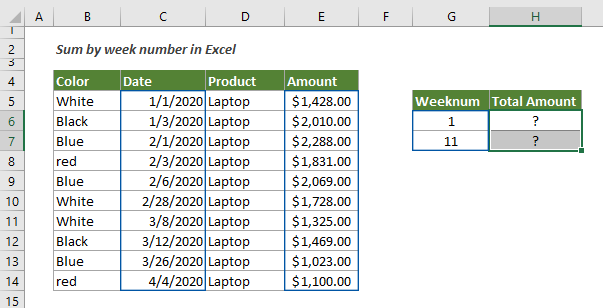Generic Formulas

=SUMIFS( value_range, helper_column_range, week_number)

=SUMPRODUCT(--(WEEKNUM(date_range+0,1)=week_number),value_range)

Arguments

Value_range: The range of values you want to sum based on the week number;
Helper_column_range: The range of week number extracted from the date;
Week_number: The week number used as criteria for summing values.

How to use this formula?

Using the SUMIFS formula and a helper column

To use the SUMIFS formula, first, you need to apply the WEEKNUM function to create a helper column to calculate the corresponding week numbers for the dates in the Date column.

=WEEKNUM (serial_num, [return_type])

The WEEKNUM function returns the week number of the given date in a year.

1. In this case, I select a cell (F5) next to the first value in the Amount column.

2. Enter the below formula into it and press the Enter key to get the week number of the first date. Select the result cell and then drag its AutoFill Handle down to get the other week numbers. See screenshot:

=WEEKNUM(C5)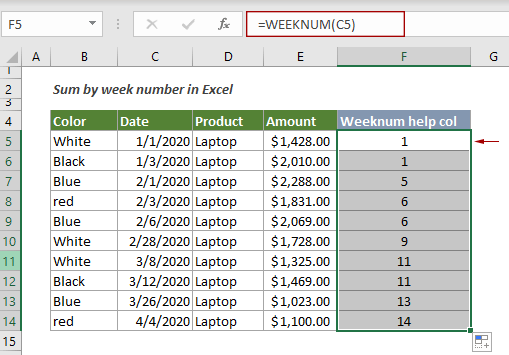3. Select a cell to output the total amount, enter the below formula into it and press the Enter key. Select this result cell, drag its AutoFill Handle down to get the other results.

=SUMIFS(\$E\$5:\$E\$14, \$F\$5:\$F\$14, H6)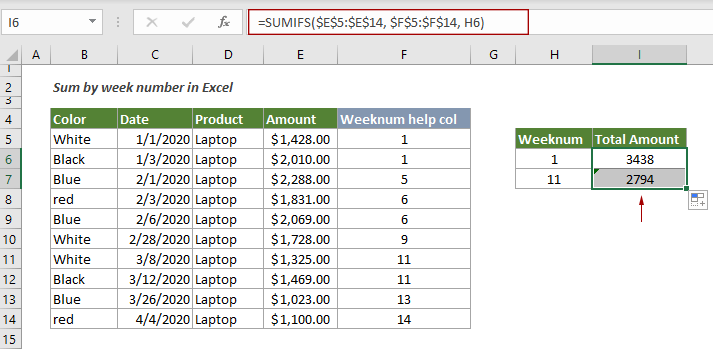Using the SUMPRODUCT formula

You don’t need to create a helper column when using the SUMPROFUCT formula to solve this problem.

1. Select a blank cell, enter the below formula into it and press the Enter key.

=SUMPRODUCT(--(WEEKNUM(\$C\$5:\$C\$14+0,1)=G6),\$E\$5:\$E\$14)

2. Select the result cell, drag its AutoFill Handle down to get the other results according to your needs.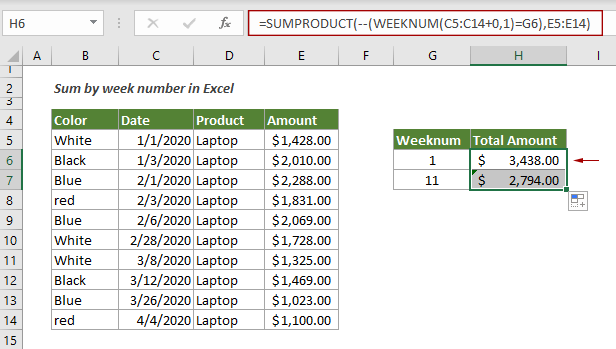Explanation of the formula

=SUMIFS(\$E\$5:\$E\$14, \$F\$5:\$F\$14, H6)

After calculating the week numbers with the WEEKNUM function, the SUMIFS function sum values in range E5:E14 if the week numbers in the helper column F5:F14 match the given week in H6.

=SUMPRODUCT(--(WEEKNUM(\$C\$5:\$C\$14+0,1)=G6),\$E\$5:\$E\$14)

WEEKNUM(\$C\$5:\$C\$14+0,1): The WEEKNUM function calculates each week number of the dates in range C5:C14, and returns an array like this: {1;1;5;6;6;9;11;11;13;14};
{1;1;5;6;6;9;11;11;13;14}=G6: Here compare each week number in the array with the given week number in G6, and returns a new array: {TRUE;TRUE;FALSE;FALSE;FALSE;FALSE;FALSE;FALSE;FALSE;FALSE};
--{TRUE;TRUE;FALSE;FALSE;FALSE;FALSE;FALSE;FALSE;FALSE;FALSE}: These two minus signs convert the “TRUE” and “FALSE” into 1s and 0s. Here you will get a new array as {1;1;0;0;0;0;0;0;0;0};
{1;1;0;0;0;0;0;0;0;0},{1428;2010;2288;1831;2069;1728;1325;1469;1023;1100}: Each pair of entries in the arrays is multiplied together and returns {1428;2010;0;0;0;0;0;0;0;0};
SUMPRODUCT({1428;2010;0;0;0;0;0;0;0;0}): The SUMPRODUCT function sums all numbers in the array and returns the final result 1428+2010=3438.

#### How to sum values by weekday in Excel?

As shown in the screenshot below, to sum values in the Amount column by the certain weekday in Excel, you can apply the SUMPRODUCT function together with the WEEKDAY function to get it done.

In this case, since we need to sum the total amount for each weekday, before applying the formula, we create a helper column with numbers 1 to 7 to separately indicate Monday to Sunday.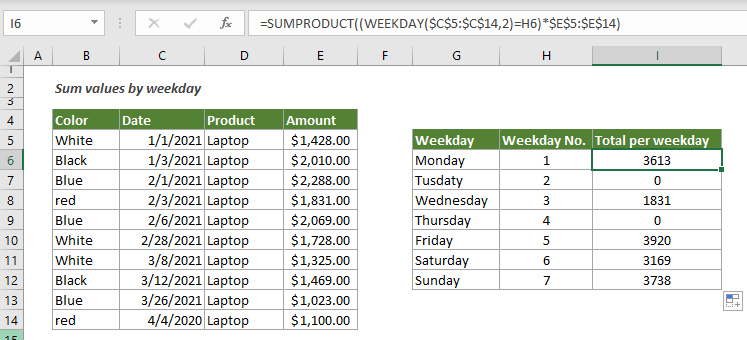Generic formula

=SUMPRODUCT((WEEKDAY(date_range,2)=weekday_number)*value_range)

Arguments

Date_range: The date range where you want to evaluate the given weekdays;
Value_range: The range of values you want to sum based on the weekday;
2: The number 2 represents numbers 1 (Monday) through 7 (Sunday);
Weekday_number: The weekday number used as criteria for summing values. It can be a cell reference or a number from 1 to 7.

How to use this formula?

1. Select a cell next to the number 1 cell. Here I select I6.

2. Copy or enter the below formula into it and press the Enter key. Select the result cell, and then drag its AutoFill Handle down to get the results for other weekdays.

=SUMPRODUCT((WEEKDAY(\$C\$5:\$C\$14,2)=H6)*\$E\$5:\$E\$14)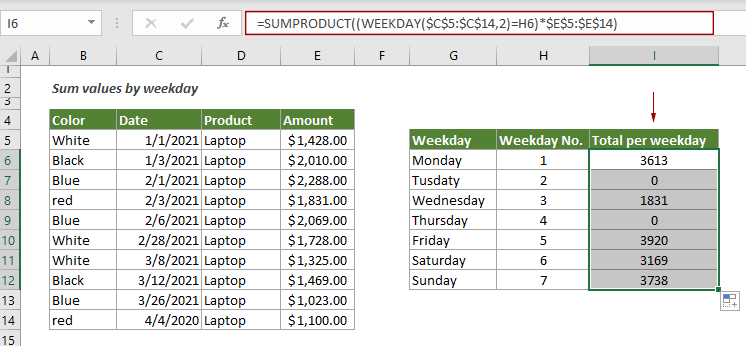Note: If you just need to sum values based on one specified weekday such as Friday, you can directly enter the number 5 in the formula as follows.

=SUMPRODUCT((WEEKDAY(\$C\$5:\$C\$14,2)=5)*\$E\$5:\$E\$14)

Explanation of the formula

=SUMPRODUCT((WEEKDAY(\$C\$5:\$C\$14,2)=H6)*\$E\$5:\$E\$14)

WEEKDAY(\$C\$5:\$C\$14,2): The WEEKDAY function returns integer numbers from 1 to 7 to represent the day of the week from Monday to Sunday in range C5:C14: {5;7;1;3;6;7;1;5;5;6};
{5;7;1;3;6;7;1;5;5;6}=H6: Here compares each day of the week in the array with the given weekday number in H6, and returns a new array: {FALSE;FALSE;TRUE;FALSE;FALSE;FALSE;TRUE;FALSE;FALSE;FALSE};
{FALSE;FALSE;TRUE;FALSE;FALSE;FALSE;TRUE;FALSE;FALSE;FALSE}*\$E\$5:\$E\$14: Here the TRUE and False are converted to 1s and 0s, and then multiplied with the values in E5:E14 to return the array: {0;0;2288;0;0;0;1325;0;0;0};
SUMPRODUCT{0;0;2288;0;0;0;1325;0;0;0}: The SUMPRODUCT function sums all numbers in the array and returns the final result 2288+1325=3613.

#### Related functions

Excel WEEKNUM function
The Excel SUMIFS function returns the week number of the given date in a year, which starts counting weeks from January 1.

Excel SUMPRODUCT function
The Excel SUMPRODUCT function can be used to multiply two or more columns or arrays together, and then get the sum of products.

Excel WEEKDAY function
The Excel EOMONTH function returns an integer number from 1 to 7 to represent the day of the week for a given date in Excel.

#### Related formulas

Sum values by month (with or without year)
This tutorial demonstrates two formulas in detail to help summing values by certain month and year or summing values by certain month ignore year in Excel.

Sum values by the week of a given date
This tutorial talks about how to sum values by the week in which a given date falls

Sum every n rows or columns in a range
This tutorial explains two formulas based on the SUM and OFFSET functions to sum every n rows or columns in a specified range in Excel.

Sum every nth row or column in a range
This tutorial is going to show you how to create a formula based on the SUMPRODUCT, MOD and COLUMN functions to sum every nth row or column in a range in Excel.

### The Best Office Productivity Tools

#### Kutools for Excel - Helps You To Stand Out From Crowd

Would you like to complete your daily work quickly and perfectly? Kutools for Excel brings 300 powerful advanced features (Combine workbooks, sum by color, split cell contents, convert date, and so on...) and save 80% time for you.

• Designed for 1500 work scenarios, helps you solve 80% Excel problems.
• Reduce thousands of keyboard and mouse clicks every day, relieve your tired eyes and hands.
• Become an Excel expert in 3 minutes. No longer need to remember any painful formulas and VBA codes.
• 30-day unlimited free trial. 60-day money back guarantee. Free upgrade and support for 2 years.#### Office Tab - Enable Tabbed Reading and Editing in Microsoft Office (include Excel)

• One second to switch between dozens of open documents!
• Reduce hundreds of mouse clicks for you every day, say goodbye to mouse hand.
• Increases your productivity by 50% when viewing and editing multiple documents.
• Brings Efficient Tabs to Office (include Excel), Just Like Chrome, Firefox, And New Internet Explorer.